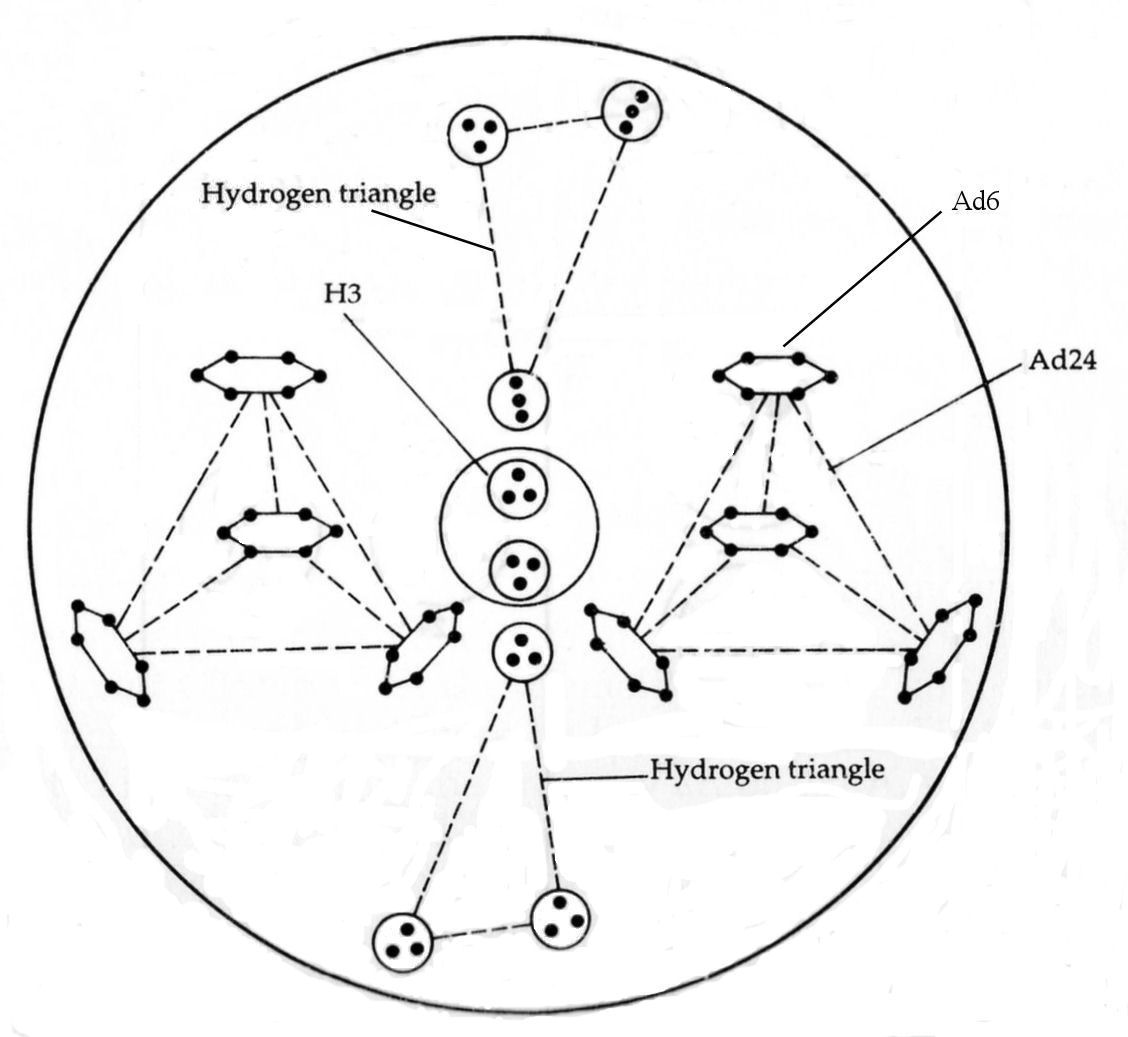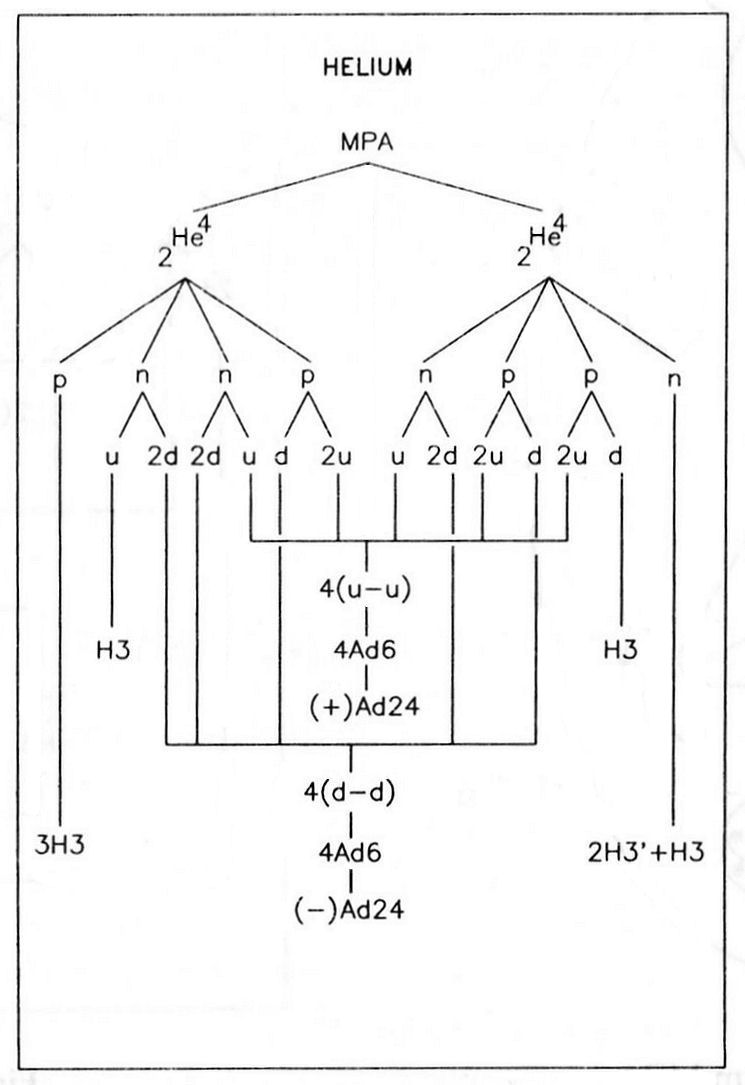The Helium MPA

The helium MPA is a sphere enclosing two separate hydrogen triangles, with a sphere between them containing two H3 triplets, and two tetrahedral arrays (Ad24) of four Ad6 groups. The Ad24 groups were reported to "revolve round an egg-shaped central body consisting of two H3 spheres, and the triangles spin on their own axes while performing a similar revolution."1 Also: "A positive tetrahedron of 4 Ad6 groups is counterbalanced by a similar tetrahedron which is negative."2

Helium MPA = 2H3 + 2Ad24 + (2H3′ + H3) + 3H3.The helium MPA. Formation of the helium MPA from two He4 nuclei.

The helium MPA is formed from two He4 nuclei, which provide 72 subquarks — the same as the number of UPAs counted in the MPA. The two hydrogen triangles are a proton and a neutron, the positive Ad24 is a bound state of four u-u diquarks, the negative Ad24 is a bound state of four d-d diquarks and the two H3 triplets are a u quark and a d quark. The quotation above differentiates between positive and negative Ad24 groups, indicating that the former is the bound state of four u-u diquarks with an electric charge of +16/3 and the latter is the bound state of four d-d diquarks with a charge of −8/3. The prediction that the two H3 triplets between the two hydrogen triangles are a positively charged u quark and a negatively charged d quark is confirmed by the statement that "in the centre of all the two groups of 3 Anu, being positive and negative, satisfy each other."3

References

1. Occult Chemistry (3rd ed.), p. 45.
2. Ibid.
3. Ibid.

Home